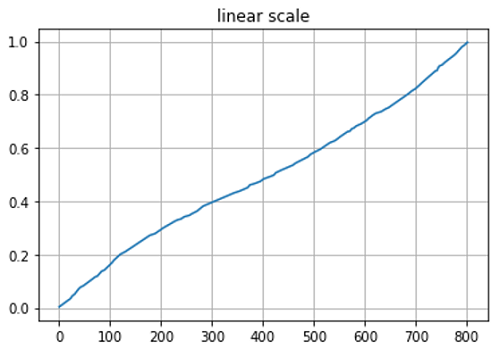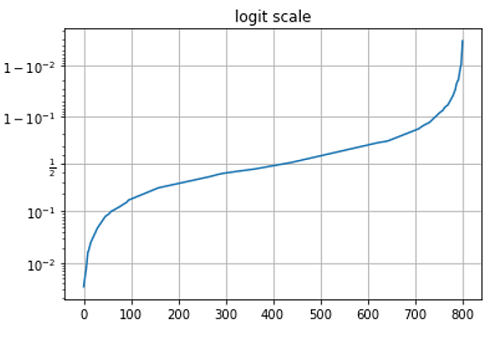# Python | Logit Scale in Plotting

In this article, we are going to learn how to change y-axis scale from linear to logit in Python plot using matplotlib?
Submitted by Anuj Singh, on August 20, 2020

When we need to plot data in logit form, then we can use an inbuilt defined function matplotlib.pyplot.yscale('logit').

We have illustrated the usage using the below example and also compared it with the linear scale in the subplot.

Illustrations:## Python code for logit scale in plotting

```import matplotlib.pyplot as plt
import numpy as np

y = np.random.normal(loc=0.5, scale=0.4, size=1000)
y = y[(y > 0) & (y < 1)]
y.sort()
x = np.arange(len(y))

plt.figure()
plt.plot(x, y)
plt.title('linear scale')
plt.grid(True)
plt.show()

plt.figure()
plt.plot(x, y)
plt.yscale('logit')
plt.title('logit scale')
plt.grid(True)
plt.show()
```

Output:

```Output is as Figure
```

Languages: » C » C++ » C++ STL » Java » Data Structure » C#.Net » Android » Kotlin » SQL
Web Technologies: » PHP » Python » JavaScript » CSS » Ajax » Node.js » Web programming/HTML
Solved programs: » C » C++ » DS » Java » C#
Aptitude que. & ans.: » C » C++ » Java » DBMS
Interview que. & ans.: » C » Embedded C » Java » SEO » HR
CS Subjects: » CS Basics » O.S. » Networks » DBMS » Embedded Systems » Cloud Computing
» Machine learning » CS Organizations » Linux » DOS
More: » Articles » Puzzles » News/Updates